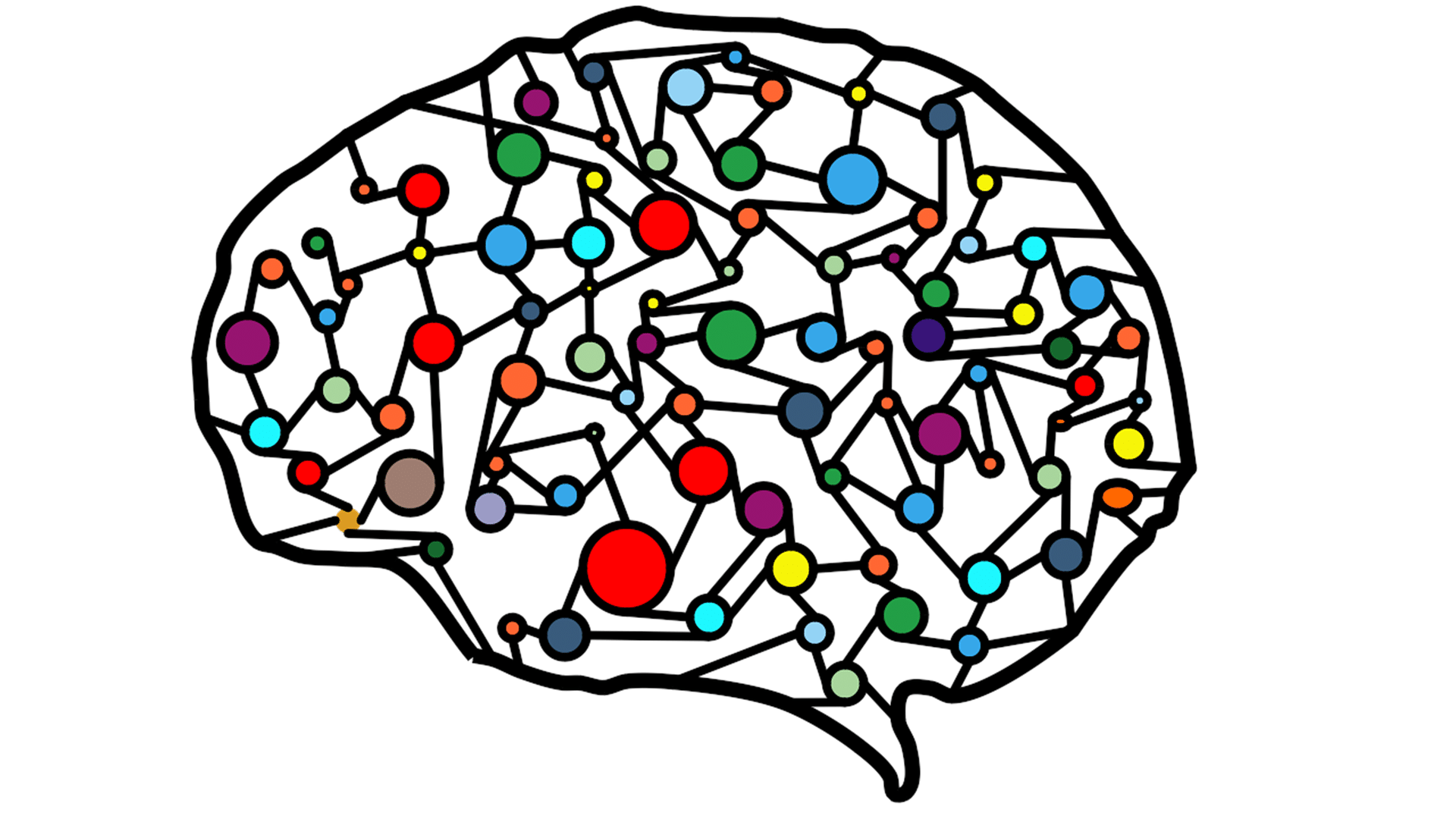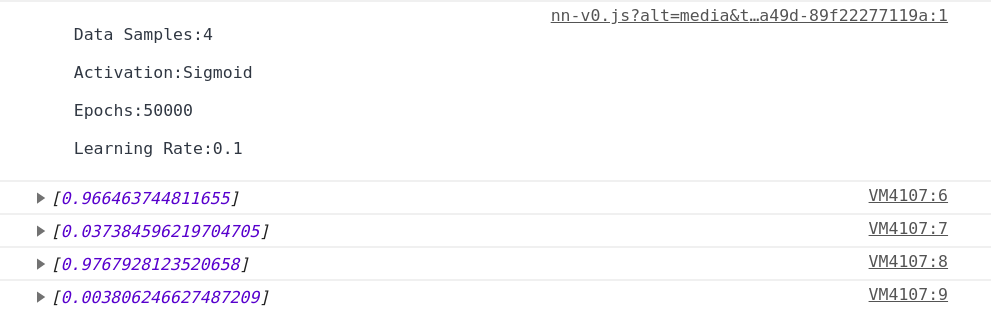# Lite Neural Networks v-0

A small lite weight JS library for your fun project

Inspried by Tensorflow.js but faster in terms of multiple Neural Networks,TF.js works superb when it comes to single Neural Network and allows hardware acceleration. But when used for creating multiple Neural Networks for applications under Genetic Algorithm or Multiple Simulation it kind of lags or consume high resources.

Lite Neural Networks Library allows you to create multiple Neural Networks and perform opeartions on them iteratively

https://cdn.jsdelivr.net/gh/suyashsonawane/Lite-Neural-Networks@latest/lib/nn-v0.js

< script src="https://cdn.jsdelivr.net/gh/suyashsonawane/Lite-Neural-Networks@latest/lib/nn-v0.js">

Creating Neural Network

// Creating a Simple Neural Network with 2 inputs 3 hidden nodes and 1 output node.
``` let nn = new NeuralNetwork([2,3,1]); ```

// Creating a Neural Network with 2 inputs 3 hidden nodes, 5 hiddden nodes, 10 hidden nodes and 10 output node.
``` let nn = new NeuralNetwork([2,3,5,10,10]); ```

Training Neural Network

// The input data is expected to be JSON object like
``` let data = [ { X: [1, 2, 3], Y: , }, , , , , { X: [7, 8, 9], Y: , }, ]; ```

// The training process can be started using the fit() function
``` let epochs = 1000 let l_rate = 0.1 // default learning rate is set to 0.01 let verbose = 1 nn.fit(data , epochs , l_rate? , verbose? ) ```

Predictions using Neural Network

// For prediction predict() function can be used
``` let X_test = [1,2,3] nn.predict(X_test) // returns 1d array ```

Smaple Program XOR Implementation

``` let data = [ { X: [0, 1], Y: , }, { X: [1, 0], Y: , }, { X: [0, 0], Y: , }, { X: [1, 1], Y: , }, ]; let nn = new NeuralNetwork([2, 5, 1]); nn.fit(data, 50000, 0.1); console.log(nn.predict([0, 1])); console.log(nn.predict([1, 1])); console.log(nn.predict([1, 0])); console.log(nn.predict([0, 0])); ```This is just a fun project in which I tried to create a simple and light weight Neural Network Library

It's actually still in development but can be used for your fun projects, I'll be pushing change logs on this website and also on my Github repo Lite Neural Network

If you find any bugs or have any ideas do create a issue on this link